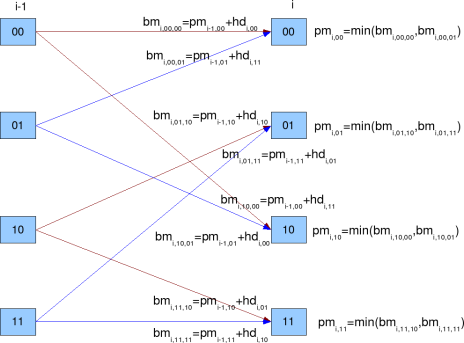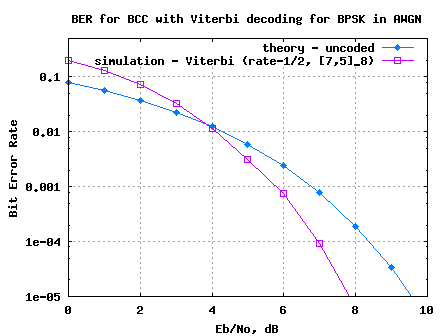- DSP log - http://www.dsplog.com -

Viterbi decoder

Posted By Krishna Sankar On January 4, 2009 @ 11:49 am In Coding | 76 Comments

Coding is a technique where redundancy is added to original bit sequence to increase the reliability of the communication. Lets discuss a simple binary convolutional coding scheme at the transmitter and the associated Viterbi (maximum likelihood) decoding scheme at the receiver.

Update: For some reason, the blog is unable to display the article which discuss both Convolutional coding and Viterbi decoding. As a work around, the article was broken upto into two posts.

This post descrbes the Viterbi decoding algorithm for a simple Binary Convolutional Code with rate 1/2, constraint length and having generator polynomial . For more details on the Binary convolutional code, please refer to the post – Convolutional code 

## Viterbi algorithm

As explained in Chapter 5.1.4 of Digital Communications by John Proakisfor optimal decoding for a modulation scheme with memory (as is the case here), if there are coded bits, we need to search from possible combinations. This becomes prohibitively complex as becomes large. However, Mr. Andrew J Viterbi  in his landmark paper Error bounds for convolutional codes and an asymptotically optimum decoding algorithm , IEEE Transactions on Information Theory 13(2):260–269, April 1967 described a scheme for reducing the complexity to more managable levels. Some of the key assumptions are as follows:

(a) As shown in Table 1 and Figure 2 (in the article Convoutional Code ) any state can be reached from only 2 possible previous state.

(b) Though we reach each state from 2 possible states, only one of the transition in valid. We can find the transition which is more likely (based on the received coded bits) and ignore the other transition.

(c) The errors in the received coded sequence are randomly distributed and the probability of error is small.

Based on the above assumptions, the decoding scheme proceed as follows: Assume that there are coded bits. Take two coded bits at a time for processing and compute Hamming distance, Branch Metric, Path Metric and Survivor Path index for times. Let be the index varying from 1 till .

#### Hamming Distance Computation

For decoding, consider two received coded bits at a time and compute the Hamming distance  between all possible combinations of two bits. The number of differing bits can be computed by XOR-ing with 00, 01, 10, 11 and then counting the number of ones.

is the number of 1′s in

is the number of 1′s in

is the number of 1′s in

is the number of 1′s in

#### Branch Metric and Path Metric Computation

As stated prior, each state can be reached from two possible states (shown by red and blue lines respectively). Branch metric is the sum of the path metric of the previous state and the hamming distance required for the transition. From the two avaiable branch metrices, the one with minimum branch metric value is chosen. This operation is also referred to as Add Compare and Select (ACS) unit.

Note:

1. Typically, Convolutional coder  always starts from State 00. The Viterbi decoder also assumes the same.

2. For index = 1, branch metric for State 00 (from State 00) branch and State 10 (from State 00) can only be computed. In this case, path metric for each state is equal to branch metric as the other branch is not valid.

3. For index = 2, branch metric for State 00 (from State 00) branch, State 01 (from State 10), State 10 (from State 00) and State 11 (from State 10) can only be computed. In this case too, path metric for each state is equal to branch metric as the other branch is not valid.

4. Starting from index =3, each state has two branches and the need to do Add Compare and Select arises.

5. Its possible that two branch metrices might have the same value. In that scenario, we can chose one among the the branches and proceed.Branch Metric and Path Metric computation for Viterbi decoder

Figure: Branch Metric and Path Metric computation for Viterbi decoder

State 00 can be reached from two branches

(a) State 00 with output 00. The branch metric for this transition is,

.

(b) State 01 with output 11. The branch metric for this transition is,

.

The path metric for state 00 is chosen based which is minimum out of the two.

The survivor path for state 00 is stored in survivor path metric.

State 01 can be reached from two branches:

(c) State 10 with output 10. The branch metric for this transition is,

(d) State 11 with output 01. The branch metric for this transition is,

The path metric for state 01 is chosen based which is minimum out of the two.

.

The survivor path for state 01 is stored in survivor path metric.

.

State 10 can be reached from two branches:

(e) State 00 with output 11. The branch metric for this transition is,

(f) State 01 with output 00. The branch metric for this transition is,

The path metric for state 10 is chosen based which is minimum out of the two.

.

The survivor path for state 10 is stored in survivor path metric.

.

State 11 can be reached from two branches:

(g) State 10 with output 01. The branch metric for this transition is,

(h) State 11 with output 10. The branch metric for this transition is,

.

The path metric for state 11 is chosen based which is minimum out of the two.

The survivor path for state 11 is stored in survivor path metric.

.

## Traceback Unit

Once the survivor path is computed times, the decoding algorithm can start trying to estimate the input sequence. Thanks to presence of tail bits (additional zeros) , it is known that the final state following Convolutional code  is State 00.

So, start from the last computed survivor path at index for State 00. From the survivor path, find the previous state corresponding to the current state. From the knowledge of current state and previous state, the input sequence can be determined (Ref: Table 2 Input given current state and previous state). Continue tracking back through the survivor path and estimate the input sequence till index = 1.

 ip, if previous state current state 00 01 10 11 00 0 0 x x 01 x x 0 0 10 1 1 x x 11 x x 1 1

Table 2: Input given current state and previous state

## Simulation Model

Octave/Matlab source code for computing the bit error rate for BPSK modulation in AWGN using the convolutional coding and Viterbi decoding is provided. Refer to the post on Bit Error Rate (BER) for BPSK modulation for signal and channel model.

Note: Since 2 coded bits are required for transmission of each data bit, the relation between coded bits to noise ratio with bits to noise ratio is

.

The simulation model performs the following:

(a) Generation of random BPSK modulated symbols +1’s and -1’s

(b) Convolutionally encode them using rate -1/2, generator polynomial [7,5] octal code

(c) Passing them through Additive White Gaussian Noise channel

(d) Hard decision demodulation on the received coded symbols

(e) Received coded bits are passed to Viterbi decoder

(f) Counting the number of errors from the output of Viterbi decoder

(g) Repeating the same for multiple Eb/No value.Figure 3: BER plot for BPSK modulation in AWGN channel with Binary Convolutional code and hard decision Viterbi decoding

## Observations

1. For lower regions, the error rate with Viterbi decoding is higher uncoded bit error rate. This is because, Viterbi decoder prefers the error to be randomly distributed for it work effectively. At lower values, there are more chances of multiple received coded bits in errors, and the Viterbi algorithm is unable to recover.

2. There can be further optimizations like – using soft decision decoding, limiting the traceback length etc. Those can be discussed in future posts.## References

Tutorial on Convolutional Coding with Viterbi Decoding  – Mr. Chip Fleming

URL to article: http://www.dsplog.com/2009/01/04/viterbi/

URLs in this post:

 Convolutional code: http://www.dsplog.com/2009/01/04/convolutional-code/

 Digital Communications by John Proakis: http://www.amazon.com/gp/redirect.html?ie=UTF8&location=http%3A%2F%2Fwww.amazon.com%2FDigital-Communications-John-Proakis%2Fdp%2F0072321113&tag=dl04-20&linkCode=ur2&camp=1789&creative=9325

 Mr. Andrew J Viterbi: http://en.wikipedia.org/wiki/Andrew_Viterbi

 Error bounds for convolutional codes and an asymptotically optimum decoding algorithm: http://ieeexplore.ieee.org/search/wrapper.jsp?arnumber=1054010

 Hamming distance: http://en.wikipedia.org/wiki/Hamming_distance

 Bit Error Rate (BER) for BPSK modulation : http://www.dsplog.com../../2007/08/05/bit-error-probability-for-bpsk-modulation/

 Matlab/Octave script for computing BER with Binary Convolutional code and Viterbi decodig for BPSK modulation in AWGN channel: http://www.dsplog.com/db-install/wp-content/uploads/2009/01/script_bpsk_ber_awgn_convlutional_code_viterbi_decode.m

 Tutorial on Convolutional Coding with Viterbi Decoding: http://home.netcom.com/~chip.f/Viterbi.html